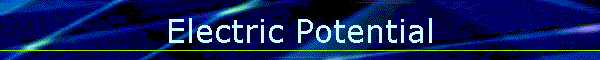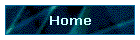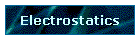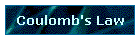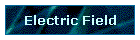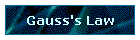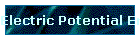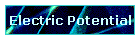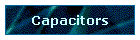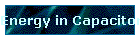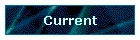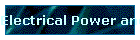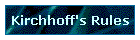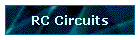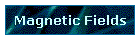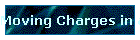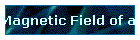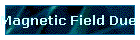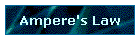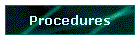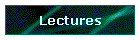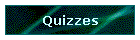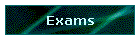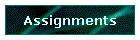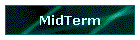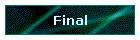Phys 4, Section 2                                                             Jan 28, 2002   Electric Potential Electric potential is defined as potential energy per unit charge. It is measured in volts. V = U/qo = kq/r                 (1 V = 1 volt = 1 J/C = 1 joule/coulomb) Here q is charge setting up the electric field and qo is a test charge being moved through the field of q. ΔV = W/qo = ∫F•ds = ∫qoE•ds/qo. If use external force per unit charge equal to -E, get negative sign ΔV = -∫E•ds   Advantage of using electric potential V instead of electric field E is that potential is a scalar field. Equipotential Surfaces Equipotential surfaces are surfaces on which the potential is everywhere the same.  No work is done in moving a charge over an equipotential surface.  The electric field at an equipotential surface must be perpendicular to the surface since otherwise there would be a component of the field and also therefore an electric force parallel to the equipotential surface.  Then work would be done in moving charges over the surface and the surface would therefore not be an equipotential surface.   Last modified on February 27, 2002